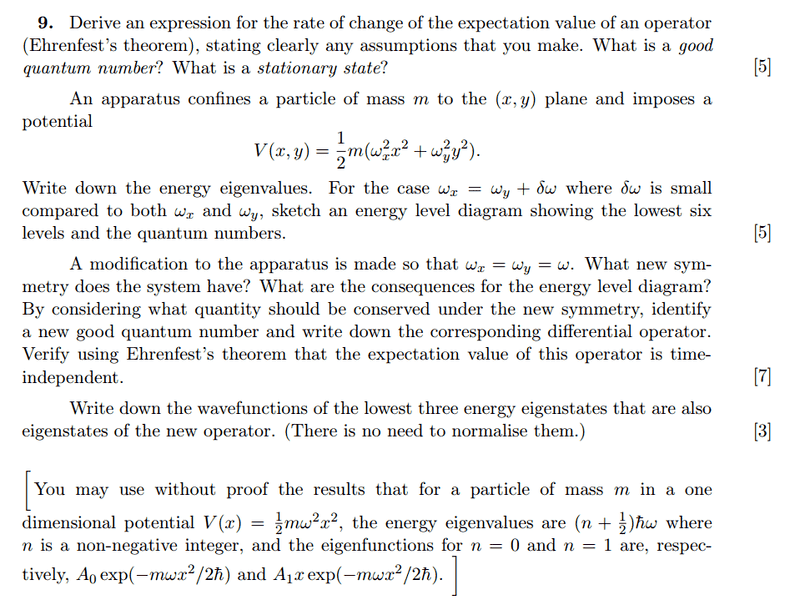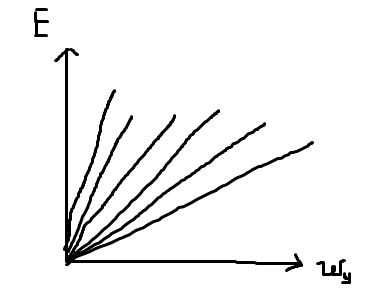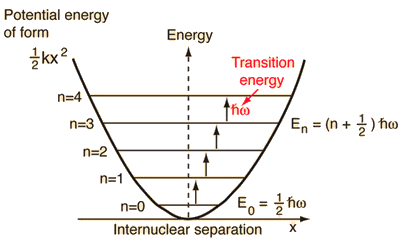# 2D Harmonic Oscillator and Ehrenfest's Theorem

## Homework StatementPart (a): Derive Ehrenfest's Theorem. What is a good quantum number?
Part (b): Write down the energy eigenvalues and sketch energy diagram showing first 6 levels.
Part (c): What's the symmetry of the new system and what happens to energy levels? Find a new good quantum number and corresponding operator. Use Ehrenfest's to show it is true.
Part (d): Write down the new wavefunctions.

## The Attempt at a Solution

Part (a)
$$\frac{d}{dt}<\psi|Q|\psi> = -<\psi|HQ|\psi> + i\hbar<\psi|\frac{dQ}{dt}|\psi> + <\psi|QH|\psi>$$
$$= <\psi|QH - HQ|\psi> + i\hbar<\psi|\frac{dQ}{dt}|\psi>$$
Assuming observable doesn't change with time:
$$= <\psi|[Q,H]|\psi>$$

If ##[Q,H] = 0##, then Q and H share a common ket ##|\psi>##such that ##Q|\psi> = q_o|\psi>##, where ##q_0## is the good quantum number.

Stationary state is when ##\frac{d<Q>}{dt} = 0##.

Part (b)

$$E = (n_x + \frac{1}{2})\hbar \omega_x + (n_y + \frac{1}{2})\hbar \omega_y$$

For ##\omega_x = \omega_y + \delta \omega##, ##E = \hbar \omega_y(n_x + n_y +1) + (n_x + \frac{1}{2})\hbar \delta \omega##.

Can I assume that energy contribution of ##\delta \omega## is small that when drawing the graph I can just ignore it?

In ascending order, the energy levels are: ##\hbar\omega_y##, ##2\hbar\omega_y##, ##3\hbar \omega_y## .... where (nx,ny): the first is nx = ny = 0, second is (0,1) or (1,0), the third is (1,1) or (2,0) or (0,2).

And how do I sketch the energy levels? Is it simply:Part (c)

There's hardly any change to the energy diagram, since we ignored the contribution due to ##\delta \omega##?

I'm guessing the symmetry is the good quantum number ##n = n_x + n_y## is conserved? What do they mean by the corresponding differential operator?

Part (d)

I have no idea why the energy eigenstates will change. Are they the same as eigenfunctions?

## Answers and Replies

Simon Bridge
Science Advisor
Homework Helper
What do they mean by the corresponding differential operator?
... the quantum number corresponds to an eigenvalue of an operator. Like the "n" number for the energy level corresponds to the eigenvalue ##E_n## of the Hamiltonian operator, which can be written in differential form as##-\frac{\hbar^2}{2m}\nabla^2##.

I have no idea why the energy eigenstates will change. Are they the same as eigenfunctions?
That's right - an eigenstate is what the eigenfunction represents.

It was probably not a good idea to ignore the ##\delta\omega## - if it has no effect, then why bother putting it in in the first place?

... the quantum number corresponds to an eigenvalue of an operator. Like the "n" number for the energy level corresponds to the eigenvalue ##E_n## of the Hamiltonian operator, which can be written in differential form as##-\frac{\hbar^2}{2m}\nabla^2##.

That's right - an eigenstate is what the eigenfunction represents.

It was probably not a good idea to ignore the ##\delta \omega## - if it has no effect, then why bother putting it in in the first place?

Ok, so I can't ignore ##\delta \omega##. So all the graphs should be slightly more steep in the plot.

For part (c), when ##\omega_x = \omega_y## The graphs become less steep, as the additional contribution due to ##delta \omega## is gone.

Is the new good quantum number ##n = n_x + n_y##? So the new differential operator is the total hamiltonian ##-\frac{\hbar^2}{2m} \nabla^2 + m\omega^2(x^2 + y^2)##?

Last edited:
Simon Bridge
Science Advisor
Homework Helper
For some reason you have plotted ##E## vs ##\omega_y##?
You also left off the quantum numbers.

I think you have been hazy about the symmetry - did you actually write down the potentials and look at them to see what the symmetry was?

For some reason you have plotted ##E## vs ##\omega_y##?
You also left off the quantum numbers.

I think you have been hazy about the symmetry - did you actually write down the potentials and look at them to see what the symmetry was?

The energy is is a function of ##\omega_y##:
$$E = \hbar \omega_y(n_x + n_y +1) + (n_x + \frac{1}{2})\hbar \delta \omega$$

For ##\omega_x = \omega_y = \omega##:

The new potential is: ##V = \frac{1}{2}m \omega^2(x^2 + y^2)##

So is the new symmetry in x and y? Do I define a new variable ##z^2 = x^2 + y^2##?

Simon Bridge
Science Advisor
Homework Helper
The energy is is a function of ##\omega_y##:
$$E = \hbar \omega_y(n_x + n_y +1) + (n_x + \frac{1}{2})\hbar \delta \omega$$
Look in your text for an energy level diagram - do the energy level lines slope like your's do?
Aside - if ##\omega_y=0## what is ##E_{n_x,n_y}##? Compare with what you sketched.

The new potential is: ##V = \frac{1}{2}m \omega^2(x^2 + y^2)##

So is the new symmetry in x and y? Do I define a new variable ##z^2 = x^2 + y^2##?
In your experience, what does ##x^2+y^2## usually equal?

Look in your text for an energy level diagram - do the energy level lines slope like your's do?

Oh the graphs are V against x, quadratic in shape.

In your experience, what does ##x^2+y^2## usually equal?

That is just the displacement, ##r^2##.

Simon Bridge
Science Advisor
Homework Helper
Oh the graphs are V against x, quadratic in shape.
That is the shape of the potential - what shape are the energy levels shown on those graphs?
Are they sloping lines? I suspect you'll find they are drawn horizontally yes?

If you look at the labels for the energy eigenvalues for each energy - how do they handle the angular frequency dependence?

That is just the displacement, ##r^2##.
... <sigh> you should be able to do this by now.

Doesn't the equation ##r^2=x^2+y^2## remind you of a geometric shape that has some special symmetry about it (that has a special name) that the equation ##r^2=ax^2+by^2## does not have (unless a=b)?

That is the shape of the potential - what shape are the energy levels shown on those graphs?
Are they sloping lines? I suspect you'll find they are drawn horizontally yes?

If you look at the labels for the energy eigenvalues for each energy - how do they handle the angular frequency dependence?

Yeah, the levels are horizontal lines, since for a given n, energy is fixed:... <sigh> you should be able to do this by now.

Doesn't the equation ##r^2=x^2+y^2## remind you of a geometric shape that has some special symmetry about it (that has a special name) that the equation ##r^2=ax^2+by^2## does not have (unless a=b)?

Yeah it's a circle, The question asks what symmetry does it have. In this case it has rotational symmetry for a given ##r^2 = x^2 + y^2##. So ##r^2## is conserved under rotational symmetry.

Thus the new good quantum number is ##n^2 = n_x^2 + n_y^2##. Consequence for the energy diagram is that now the energy levels of x and y collapse together. They were once separated by a small perturbation (In this sense, the perturbation "lifts" degeneracy).

Is the differential operator the angular part of the laplacian? i.e. ##L^2_{(\theta)} = \frac{1}{r}\frac{\partial}{\partial r}\left(r \frac{\partial}{\partial r}\right) + \frac{1}{r^2}\frac{\partial^2}{\partial \theta^2}##

To show it is time independent, I have to show ##[L^2_{(\theta)} , \hat H] = [L^2_{(\theta)} , \frac{p^2}{2m} + V_{(x,y)}] = [L^2_{(\theta, \phi)} , \frac{p^2}{2m} + \frac{1}{2}m\omega^2r^2] = 0 ##.

Since ##p## is always radial, and ##V = f(r)##, thus the commutator is zero.

Part (d)
Since ##L^2## commutes with ##\hat H##, they both share common eigenstates.

$$u_n = u_xu_y$$

So:
$$u_0 = u_{0x}u_{0y} = A *exp(-\frac{m\omega(x^2+y^2)}{2\hbar^2})$$

$$u_1 = u_{1x}u_{1y} = A_1 xy *exp(-\frac{m\omega(x^2+y^2)}{2\hbar^2})$$

$$u_2 = u_{2x}u_{2y}$$

Is there a way to get the n = 2 state without solving the differential equation and using lowering/raising operators?

Last edited:
Simon Bridge
Science Advisor
Homework Helper
Energy level diagrams:
Notice how the angular frequency of the energy eigenvalues is treated?
They just put it in the expression for the value.

I think this is the sort of diagram you are expected to draw.
But it is your course and your course notes may have included diagrams like what you drew calling themselves energy-level diagrams ... but that would not be the usual convention.

Perturbation:
Have you recently covered perturbation theory?

Yah - the effect of the small ##\delta\omega## is to slightly lift the energy levels - it also means that if ##\omega=0## the energy eigenvalue is still not zero. The graph of E vs ω should have a "y-intercept". It wasn't the slope that changed.

Symmetry:
if you have a circle, then the symmetry is called "circular" or "rotational".
The small perturbation before gives you elliptical symmetry.

... probably not literal circles or ellipses: you can plot the density functions and see the actual shape.

Anyway: relate it to physics - what physical properties are conserved in rotating systems?
That should help you understand the commutator :) (i.e. what does L^2 correspond to?)

Is there a way to get the n = 2 state without solving the differential equation and using lowering/raising operators?
... um - probably.
The thing about these operators is that someone had to solve the DE at some time, you just get to exploit the fact that you already know the answer. You can have a go working it out if you like.

My main concern with your reasoning above is that it is very abstract and algebraic - you are relying too much on knowing the equations and not using your physics understanding enough, or maybe you are just not putting your understanding on display enough.

You are allowed to say that ##r^2=x^2+y^2## is the equation of a circle!

You want to show off your understanding - this is where the extra marks are hiding.
If you don't show it, the marker will assume you don't have it and withhold those marks.
Showing it, otoh, can get you marks that you may not explicitly have earned ;) you can get the benefit of any doubts only if you look like you understand the physics.

It also streamlines getting help here ;)

Last edited:
Energy level diagrams:
Notice how the angular frequency of the energy eigenvalues is treated?
They just put it in the expression for the value.

I think this is the sort of diagram you are expected to draw.
But it is your course and your course notes may have included diagrams like what you drew calling themselves energy-level diagrams ... but that would not be the usual convention.

Perturbation:
Have you recently covered perturbation theory?

Yah - the effect of the small ##\delta\omega## is to slightly lift the energy levels - it also means that if ##\omega=0## the energy eigenvalue is still not zero. The graph of E vs ω should have a "y-intercept". It wasn't the slope that changed.

Symmetry:
if you have a circle, then the symmetry is called "circular" or "rotational".
The small perturbation before gives you elliptical symmetry.

... probably not literal circles or ellipses: you can plot the density functions and see the actual shape.

Anyway: relate it to physics - what physical properties are conserved in rotating systems?
That should help you understand the commutator :) (i.e. what does L^2 correspond to?)

... um - probably.
The thing about these operators is that someone had to solve the DE at some time, you just get to exploit the fact that you already know the answer. You can have a go working it out if you like.

My main concern with your reasoning above is that it is very abstract and algebraic - you are relying too much on knowing the equations and not using your physics understanding enough, or maybe you are just not putting your understanding on display enough.

You are allowed to say that ##r^2=x^2+y^2## is the equation of a circle!

You want to show off your understanding - this is where the extra marks are hiding.
If you don't show it, the marker will assume you don't have it and withhold those marks.
Showing it, otoh, can get you marks that you may not explicitly have earned ;) you can get the benefit of any doubts only if you look like you understand the physics.

It also streamlines getting help here ;)

That is great advice. By rotating the systems, things like spin orientation and energy are unchanged. ##L^2## corresponds to the total angular momentum operator, whose expected value is the expected total angular momentum. Rotating the system doesn't change the total angular momentum, so therefore d<L2>/dt = 0 (to show using ehrenfest)

Simon Bridge
Science Advisor
Homework Helper
There you go - and doesn't it feel better to say it like that?

You can get away with drawing these parallels with classical physics because classical physics is what happens to the expectation values. so QM <p> is Newtonian p etc.

We say: classical physics is what happens on average.

Last edited: